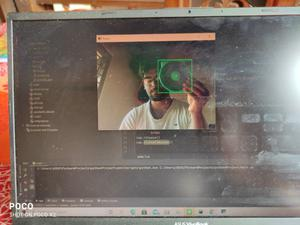# Automating Scrolling using Python-Opencv by Color Detection

• Last Updated : 13 Jan, 2021

Prerequisites:

It is possible to perform actions without actually giving any input through touchpad or mouse. This article discusses how this can be done using opencv module. Here we will use color detection to scroll screen. When a certain color is detected by the program during execution the screen starts to scroll on its own.

Attention geek! Strengthen your foundations with the Python Programming Foundation Course and learn the basics.

To begin with, your interview preparations Enhance your Data Structures concepts with the Python DS Course. And to begin with your Machine Learning Journey, join the Machine Learning - Basic Level Course

### Approach

• Import module
• Use cv2 to capture video, here to use default webcam use 0, and for any other cam use 1.
• Read the captured video and store the video frame in a variable
• Get every color of the frame.
• Create a mask of the color required to take as input to scroll using their acceptable color ranges. Here it is taken as green.
• Get contours and hierarchy from mask
• Pass contours using for loop and calculate the area.
• Add scroll mechanism when required color is detected(Green here).
• Show the frame using cv2.imshow()and pass the frame name and the frame variable to show every captured frame, put the frame capture process in a while loop. To come out of the process use a wait key and break statement.
• Then stop the window of webcam.

Below is the implementation.

## Python3

 `import` `cv2``import` `numpy as np``import` `pyautogui`` ` `low_green ``=` `np.array([``25``, ``52``, ``72``])``high_green ``=` `np.array([``102``, ``255``, ``255``])`` ` `cap ``=` `cv2.VideoCapture(``0``)`` ` `prev_y ``=` `0`` ` `while` `True``:``    ``ret, frame ``=` `cap.read()``    ``hsv ``=` `cv2.cvtColor(frame, cv2.COLOR_BGR2HSV)``    ``mask ``=` `cv2.inRange(hsv, low_green, high_green)``    ``contours, hierarchy ``=` `cv2.findContours(``        ``mask, cv2.RETR_EXTERNAL, cv2.CHAIN_APPROX_SIMPLE)`` ` `    ``for` `i ``in` `contours:``        ``area ``=` `cv2.contourArea(i)``        ``if` `area > ``1000``:``            ``x, y, w, h ``=` `cv2.boundingRect(i)``            ``cv2.rectangle(frame, (x, y), (x``+``w, y``+``h), (``0``, ``255``, ``0``), ``2``)``            ``if` `y < prev_y:``                ``pyautogui.press(``'space'``)``            ``prev_y ``=` `y``    ``cv2.imshow(``'frame'``, frame)``    ``if` `cv2.waitKey(``1``) ``=``=` `ord``(``'q'``):``        ``break`` ` `cap.release()``cap.closeAllWindow()`

Input:Detecting green color

My Personal Notes arrow_drop_up